# Types of Triangles

Go back to  'Triangles'

 1 Introduction to Triangles 2 How many sides does a Triangle have? 3 Types of Triangles 4 Angles of a Triangle 5 Classification of Triangles 6 Thinking out of the Box! 7 Solved Examples and Problems 8 Challenging Questions 9 Practice Questions 10 Important notes on Types of Triangles 11 Maths Olympiad Sample Papers 12 Frequently Asked Questions (FAQs)

We at Cuemath believe that Math is a life skill. Our Math Experts focus on the “Why” behind the “What.” Students can explore from a huge range of interactive worksheets, visuals, simulations, practice tests, and more to understand a concept in depth.

Book a FREE trial class today! and experience Cuemath’s LIVE Online Class with your child.

## Introduction to Triangles

Triangles are all around us.

If you observe a half sandwich, a sign board, nachos, and so on, you will see that they are all shaped like triangles.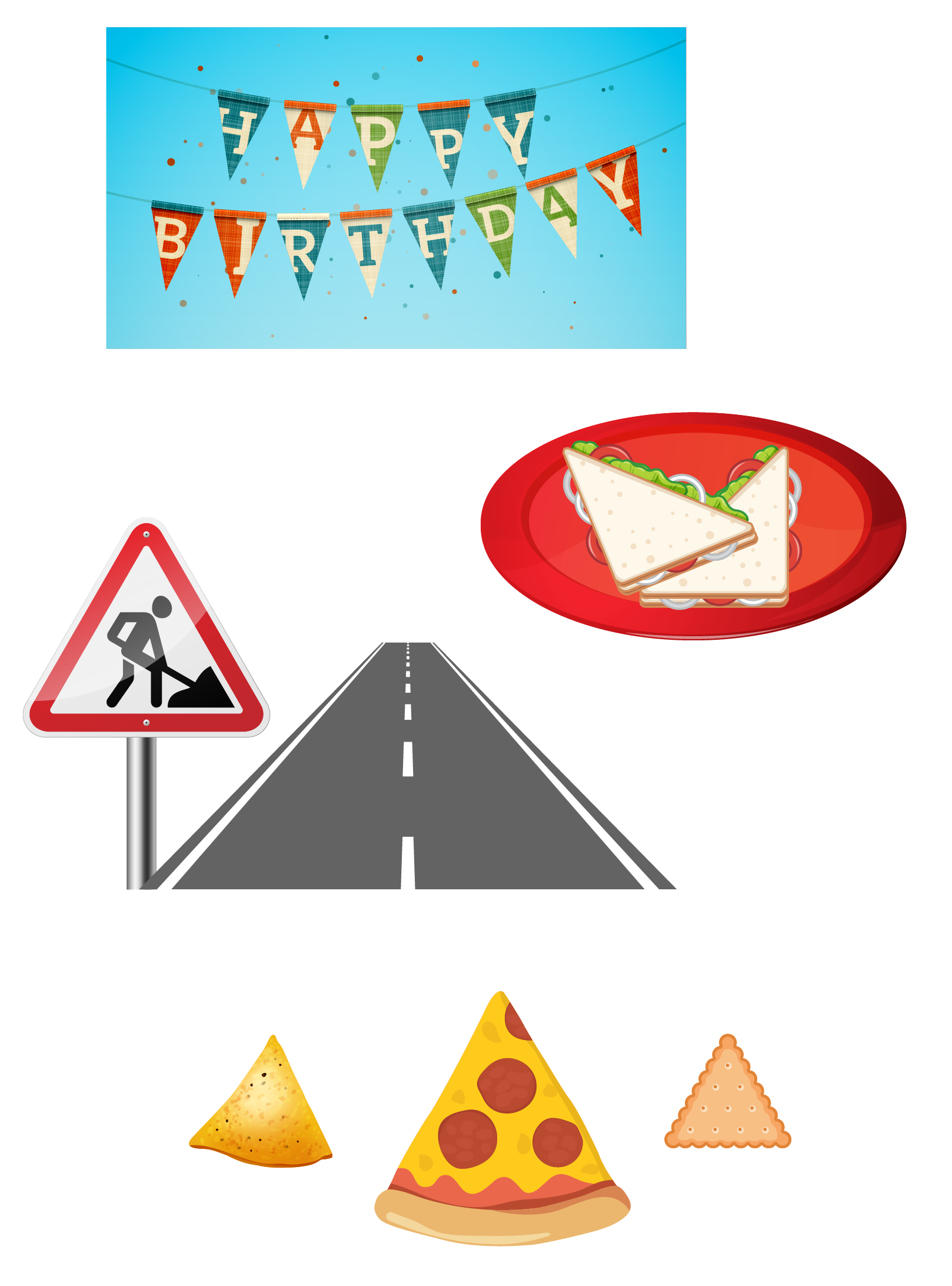Let us explore and understand triangles and their properties, and the various types of triangles.

## How many sides does a Triangle have?

Triangles are closed shapes made with 3 line segments.

The line segments which form the triangle are called its sides or edges.

The point where two sides meet is called the vertex.

An angle is formed at the vertex.

A triangle has:

• 3 sides,
• 3 angles, and
• 3 vertices (plural for vertex).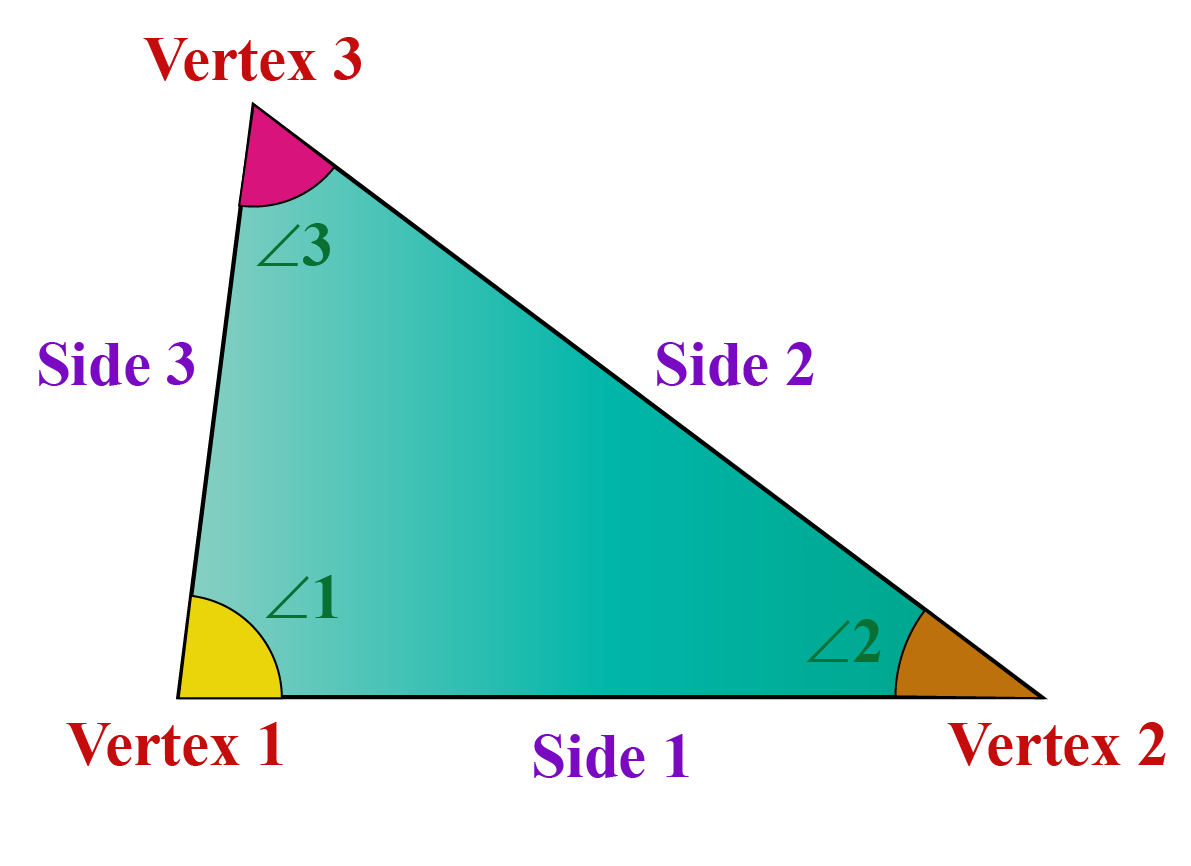Vertices are represented using upper-case alphabets.

Consider the triangle shown below.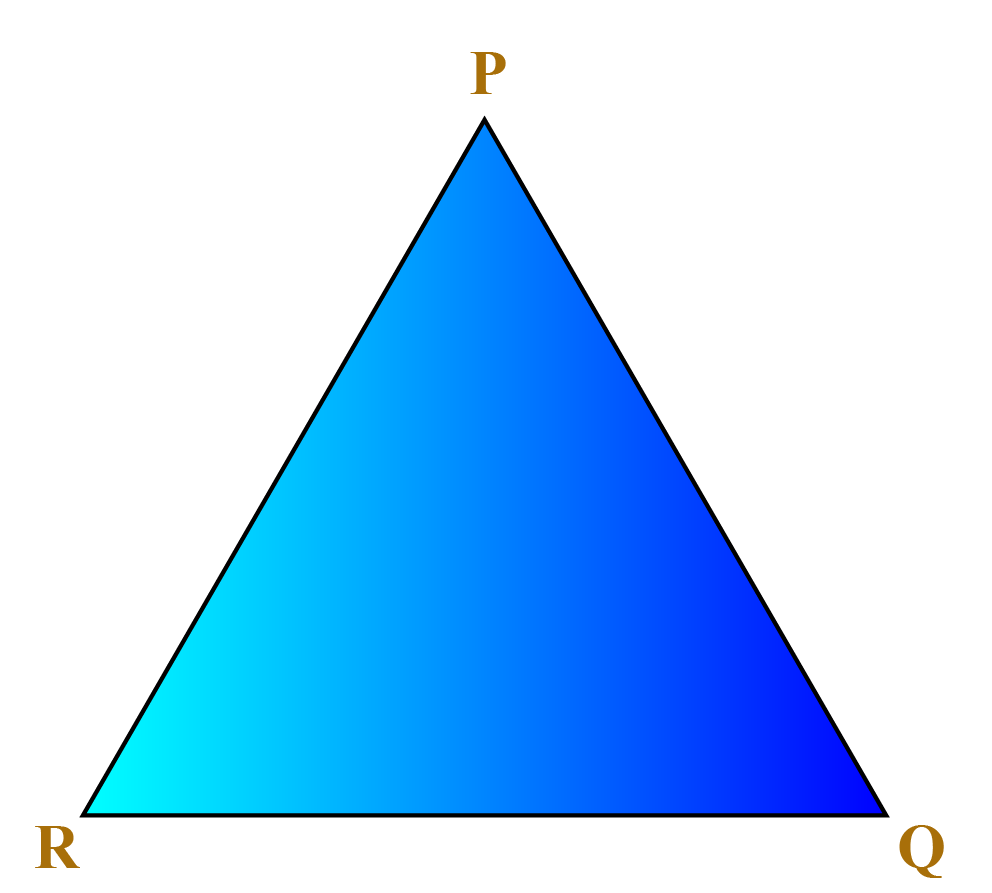The vertices of the triangle are P, Q and R.

The angles formed at these vertices are $$\angle \text{P} , \angle \text{Q} , \angle \text{R}$$

The sides of the triangle are PR, RQ and QP.

The triangle with the 3 vertices PQR is denoted as △PQR.

The 3 angles and the 3 sides are the elements of a triangle.

## Types of Triangles

A triangle has 3 sides and 3 angles.

### Types of Triangles based on the Sides

When all 3 sides of a triangle are equal, we call it an equilateral triangle.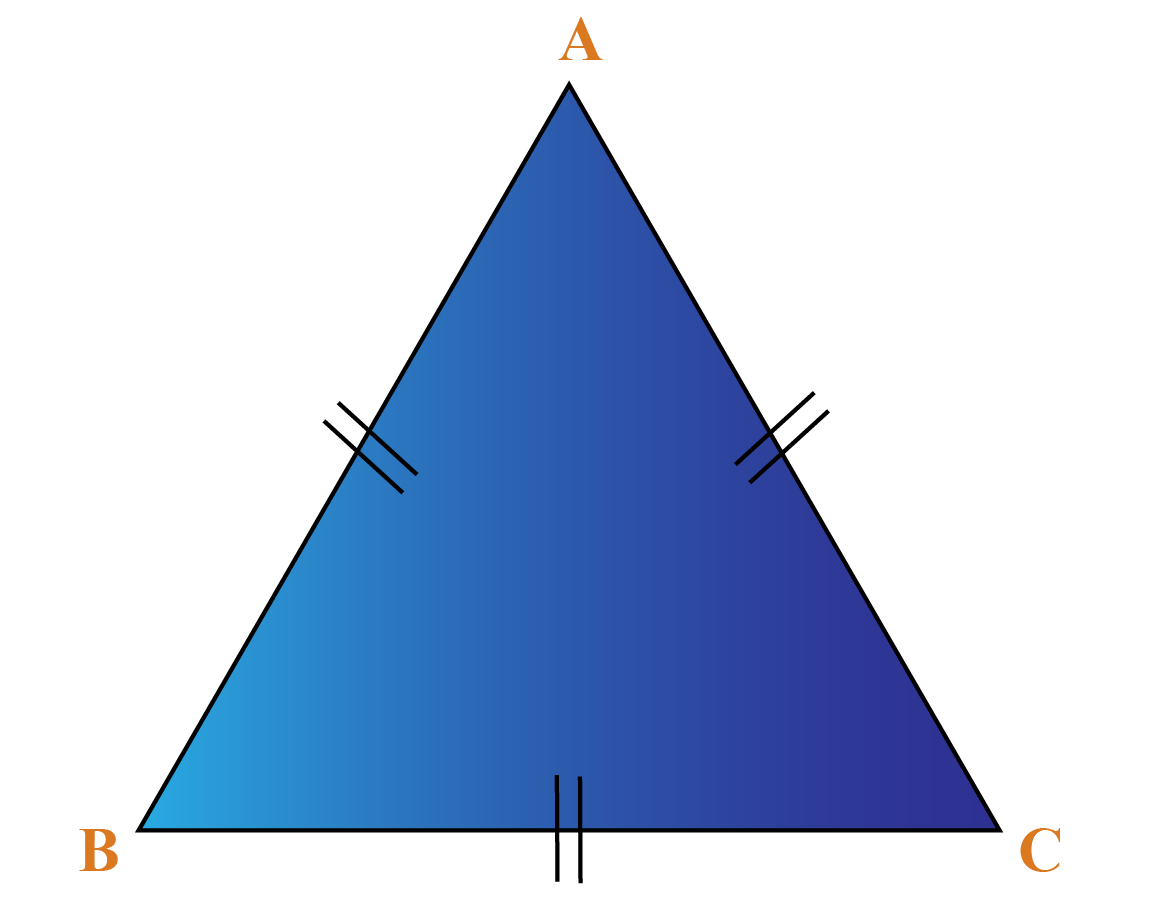The symbol || on each side denote that the sides are equal.

When 2 sides of a triangle are equal, we call it an isosceles triangle.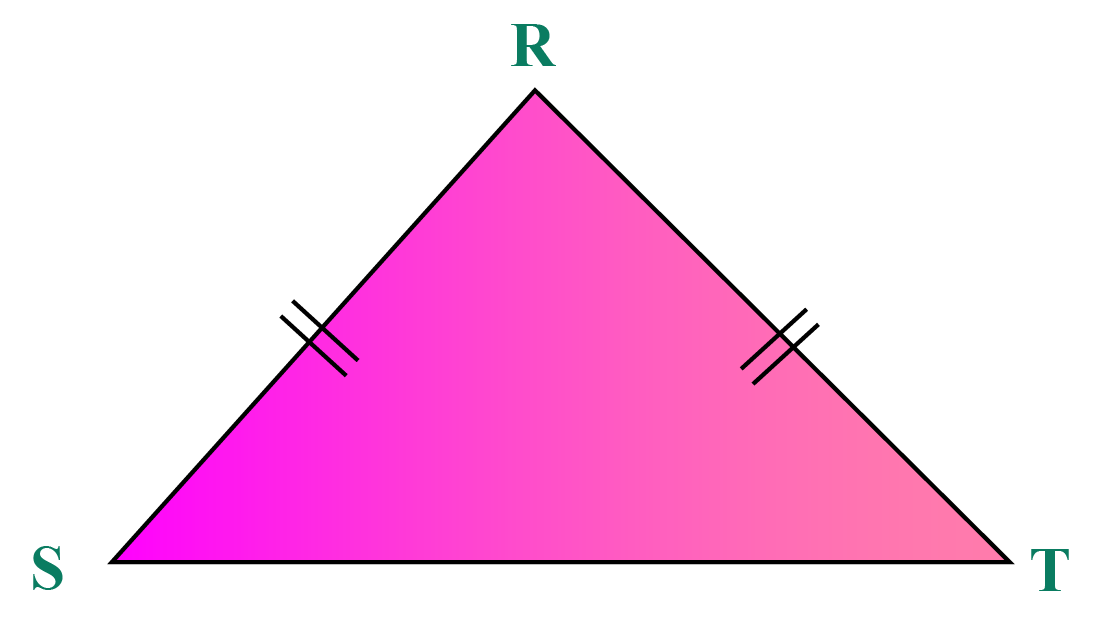In the triangle above, you can observe that there are only 2 sides with the symbol || marked on them.

When none of the sides of a triangle are equal, we call it a scalene triangle.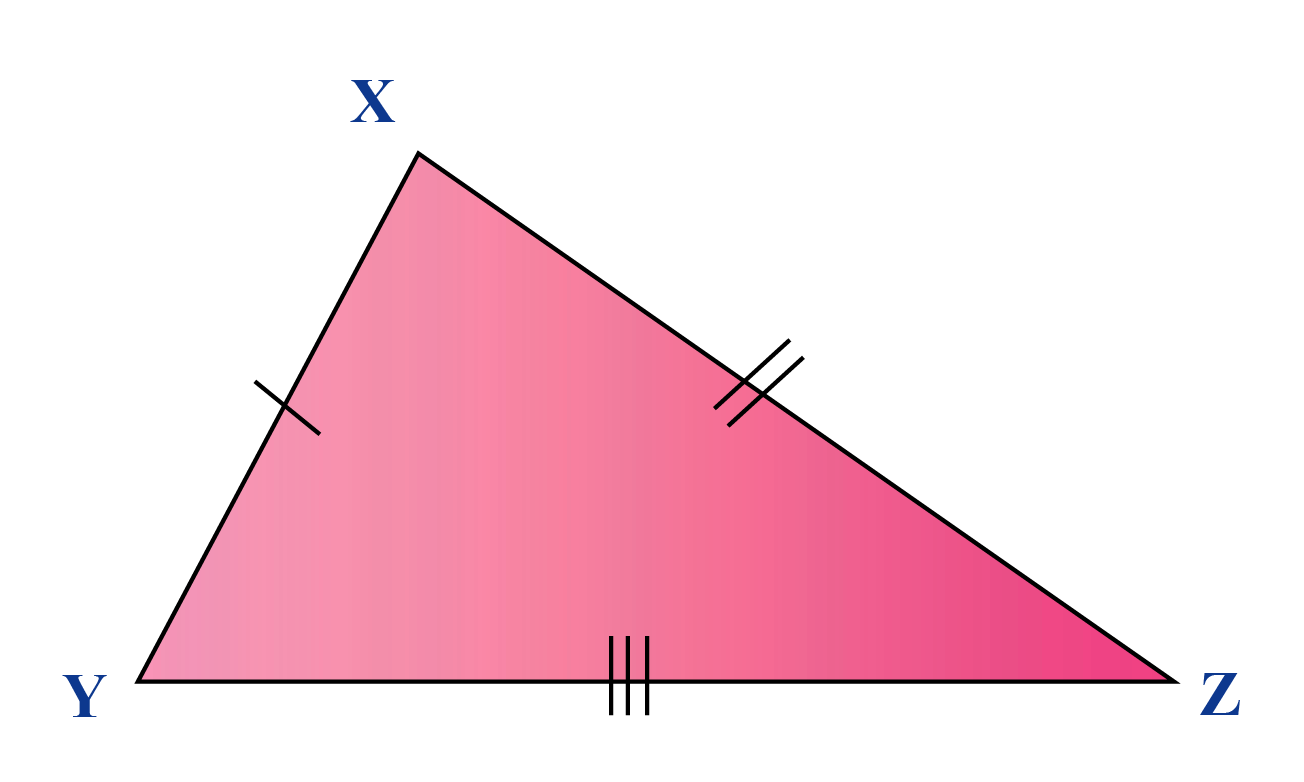In the above triangle, you can observe that all 3 symbols on each side are different which denote that all 3 sides are unequal.

### Types of Triangles based on the Angles

When all three angles of a triangle are acute angles, we call it an acute-angled triangle or simply acute triangle.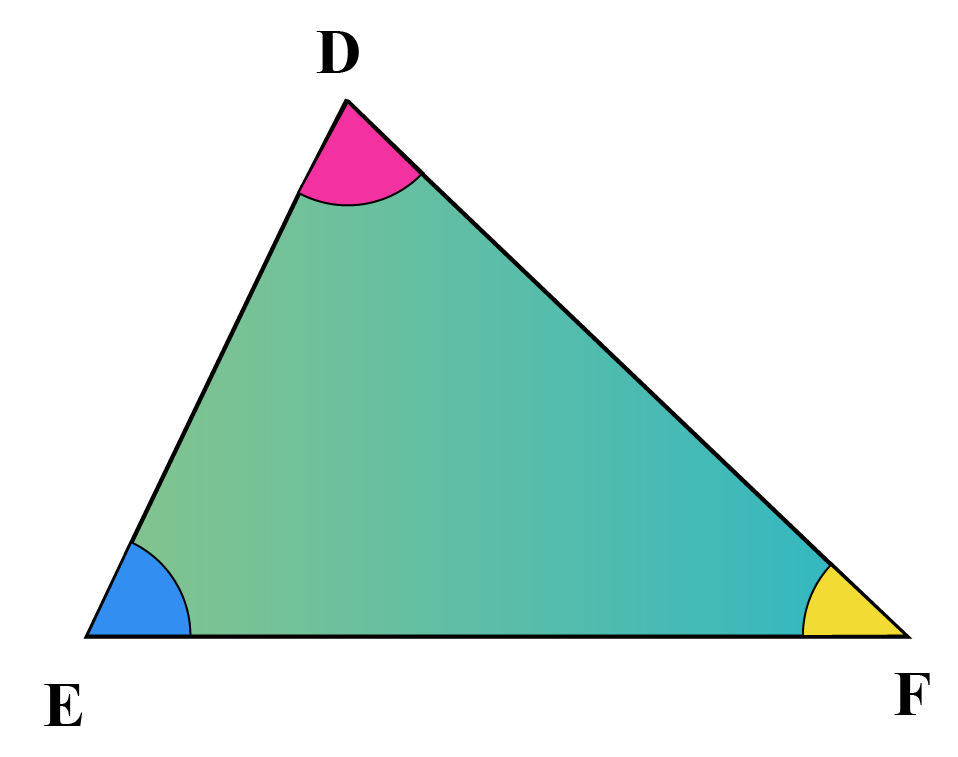When one of the angles in a triangle is right angle i.e. 90$$^\circ$$, we call it a right-angled triangle or simply Right triangle.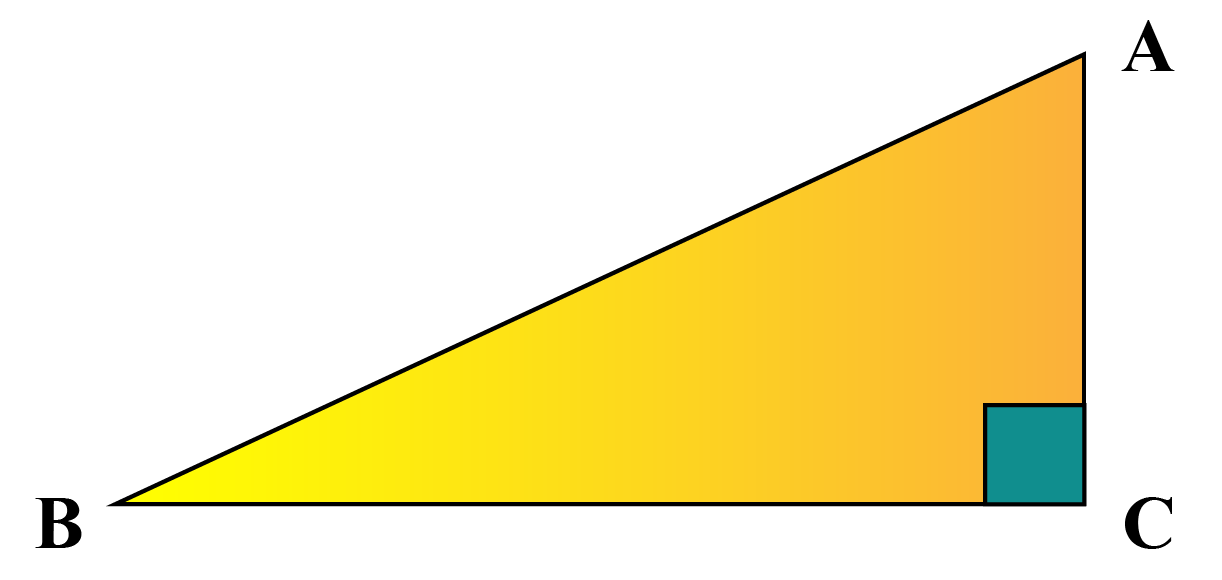In the above triangle, observe the symbol marked at vertex C.

This denotes a right angle i.e, 90$$^\circ$$

Thus, the triangle is a right-angled triangle.

When one of the angles in a triangle is an obtuse angle, we call it an obtuse-angled triangle or simply obtuse triangle.

The following triangle is an obtuse-angled triangle.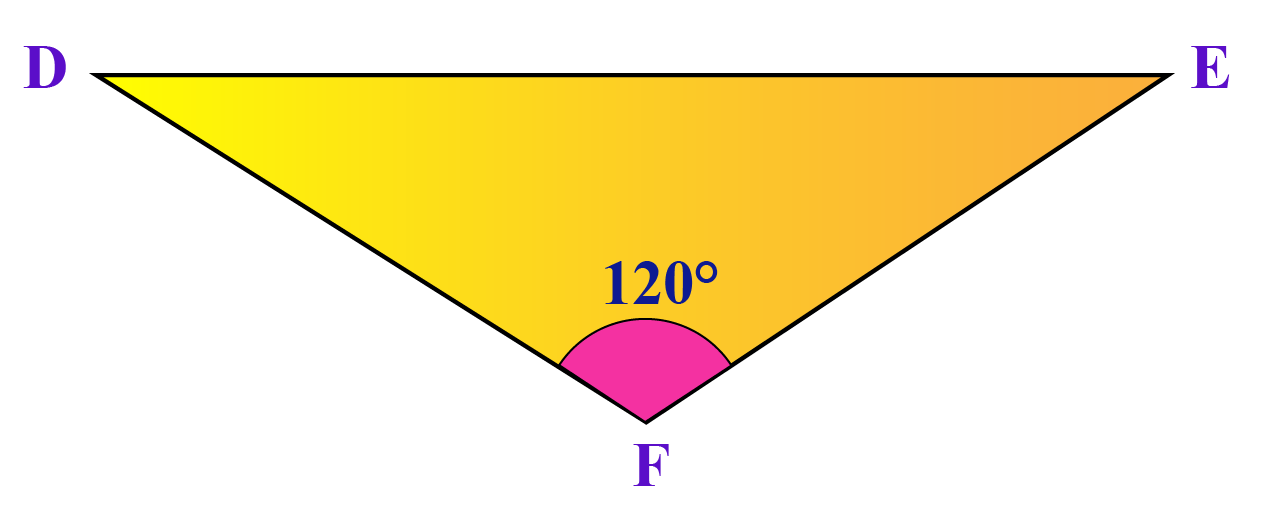## Angles of a Triangle

We know that 3 angles are formed at the 3 vertices of a triangle.

These angles are called internal angles or interior angles of the triangle.

The 3 internal angles of a triangle always add up to 180$$^\circ$$

Let us understand this statement using the following figure.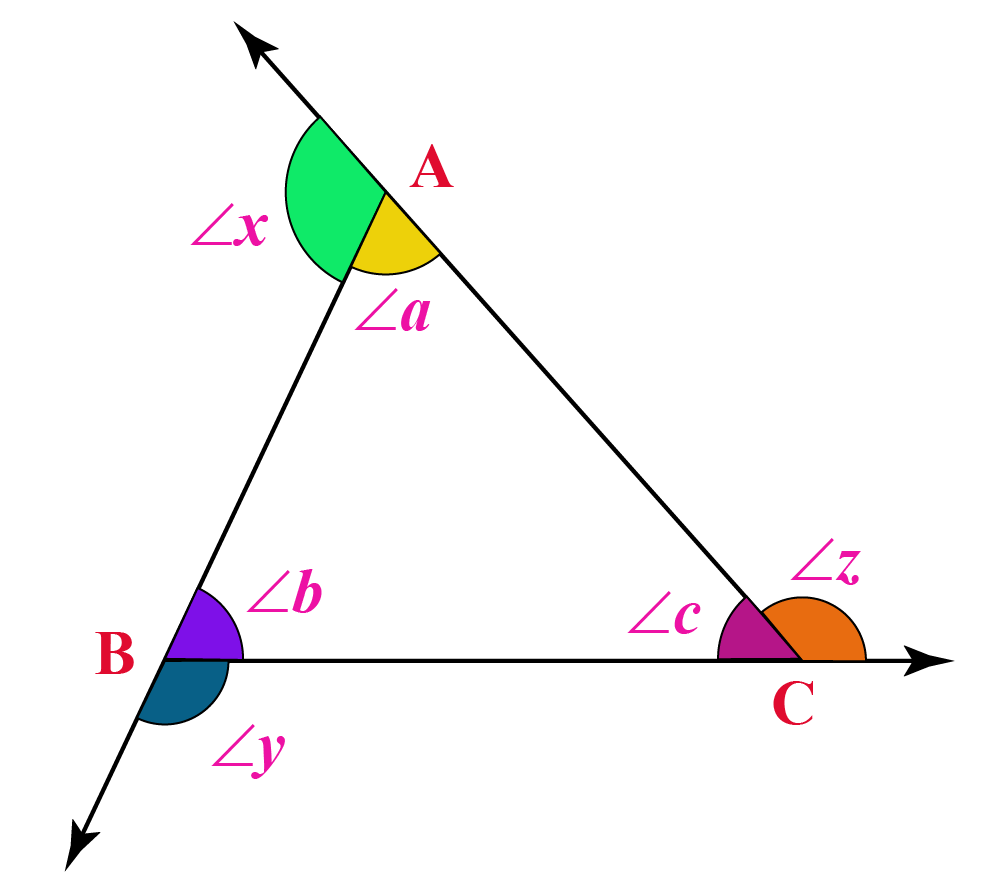In the given triangle, the line segments at each vertex are extended.

$$\angle a\: , \angle b \:\text{and}\: \angle c$$ are interior angles and they add up to 180 degrees.

$$\angle a + \angle b + \angle c = 180 ^\circ$$

$$\angle x \:,\: \angle y \: \text{and} \: \angle z$$ are exterior angles.

The internal angle and the external angle at a vertex form a linear pair .i.e they add up to 180$$^\circ$$

$$\angle b +\angle y= 180^\circ$$

Can you identify the other two linear pairs?

## Classification of Triangles

There are 3 types of triangles based on angles and 3 types of triangles based on sides.

Now let us combine them and understand the classification of triangles.

### Equilateral or Equiangular Triangle

When all sides and angles of a triangle are equal, it is called an equilateral or equiangular triangle.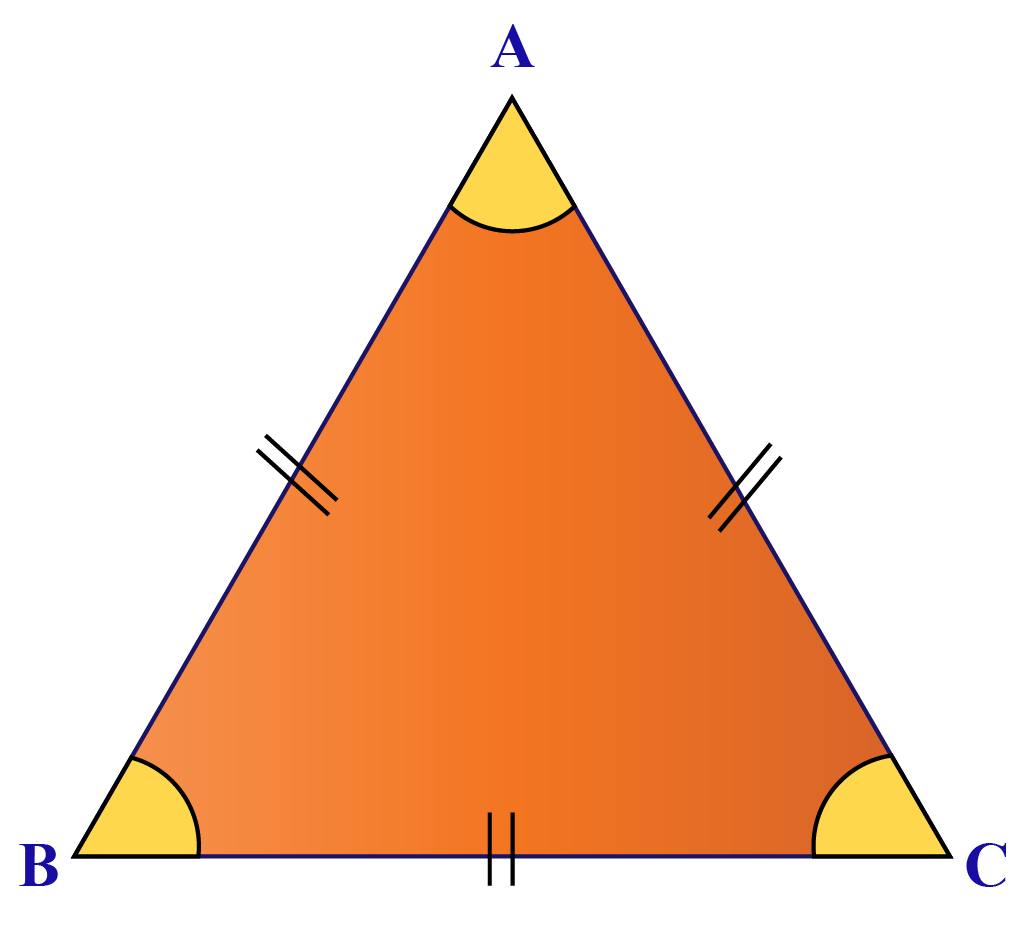### Right Isosceles Triangle

A triangle in which 2 sides are equal and one angle is 90$$^\circ$$ is called a right isosceles triangle.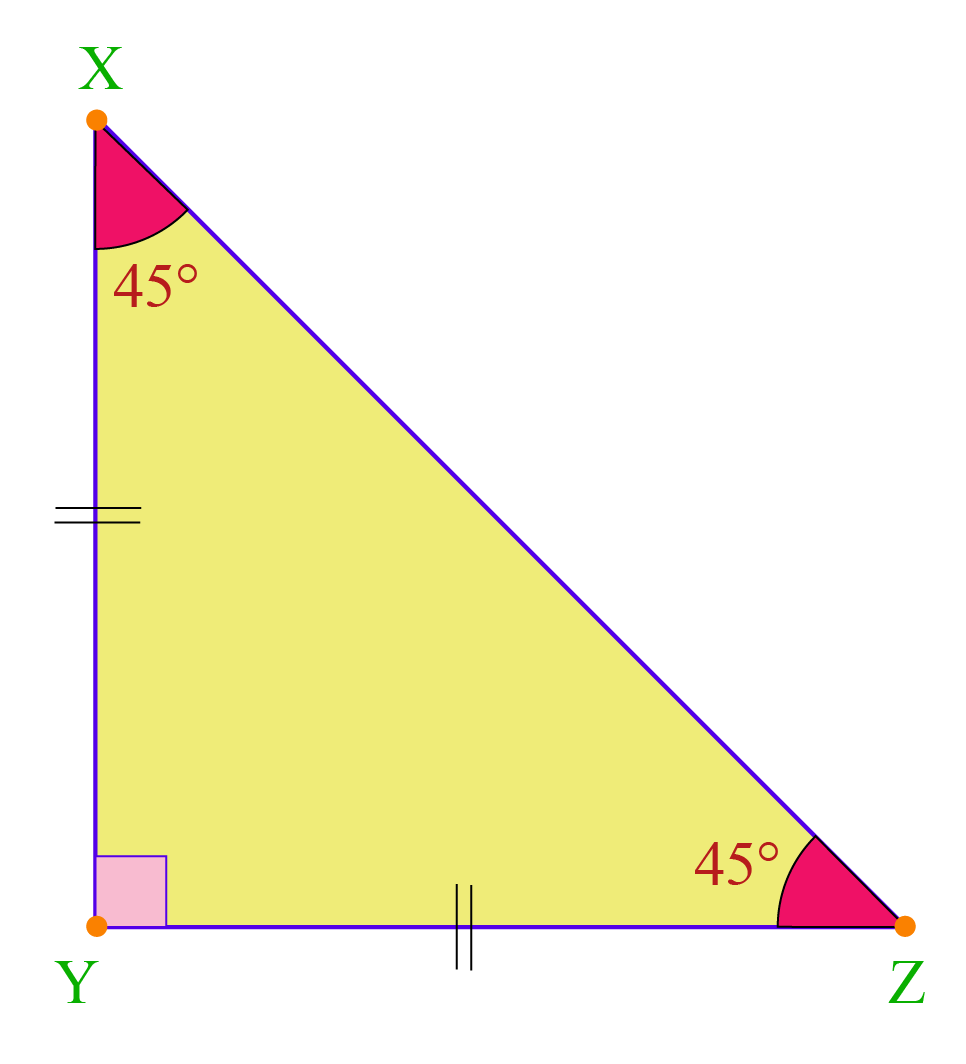### Obtuse Isosceles Triangle

A triangle in which 2 sides are equal and one angle is an obtuse angle, is called an obtuse isosceles triangle.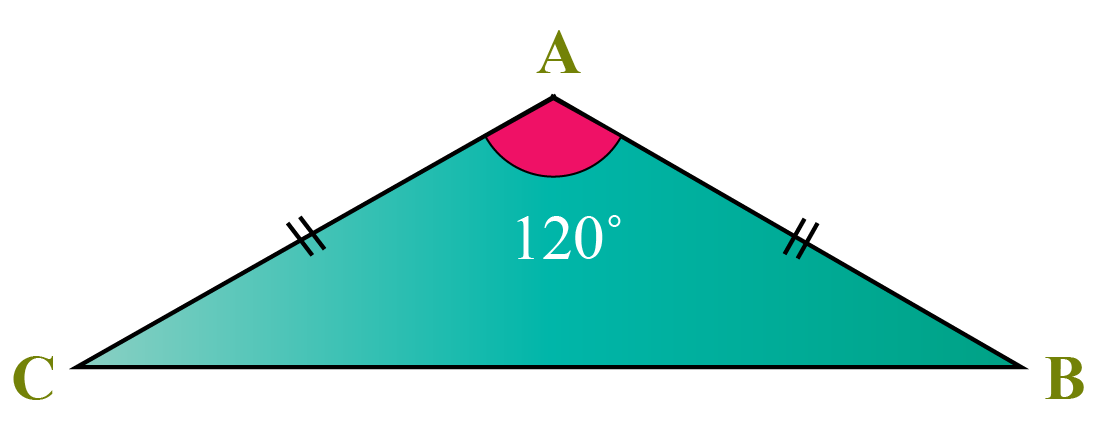### Acute Isosceles Triangle

A triangle in which all 3 angles are acute angles and 2 sides measure the same is called an acute isosceles triangle.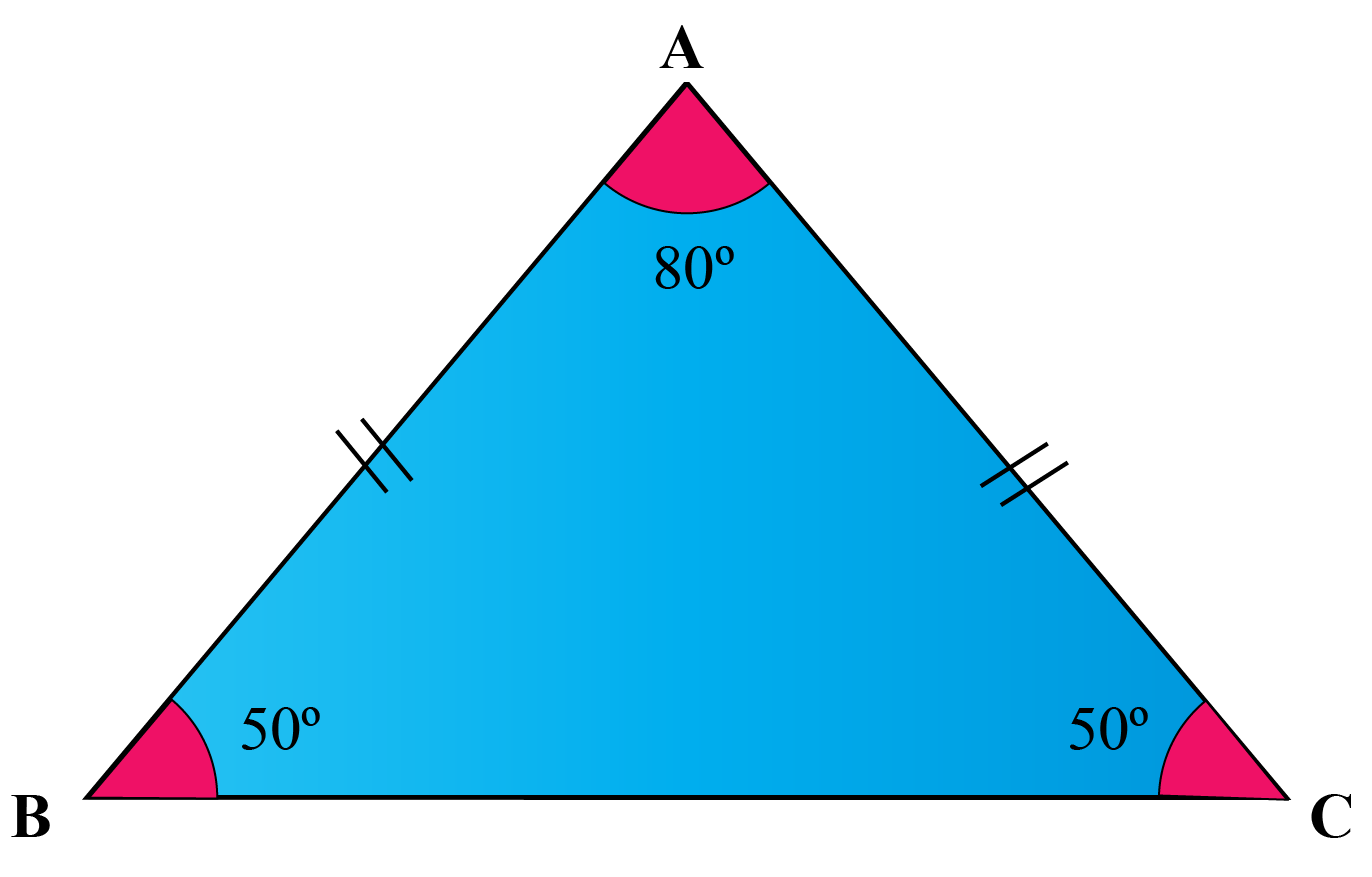### Right Scalene Triangle

A triangle in which all 3 sides are unequal and any one angle is a right angle is called a right scalene triangle.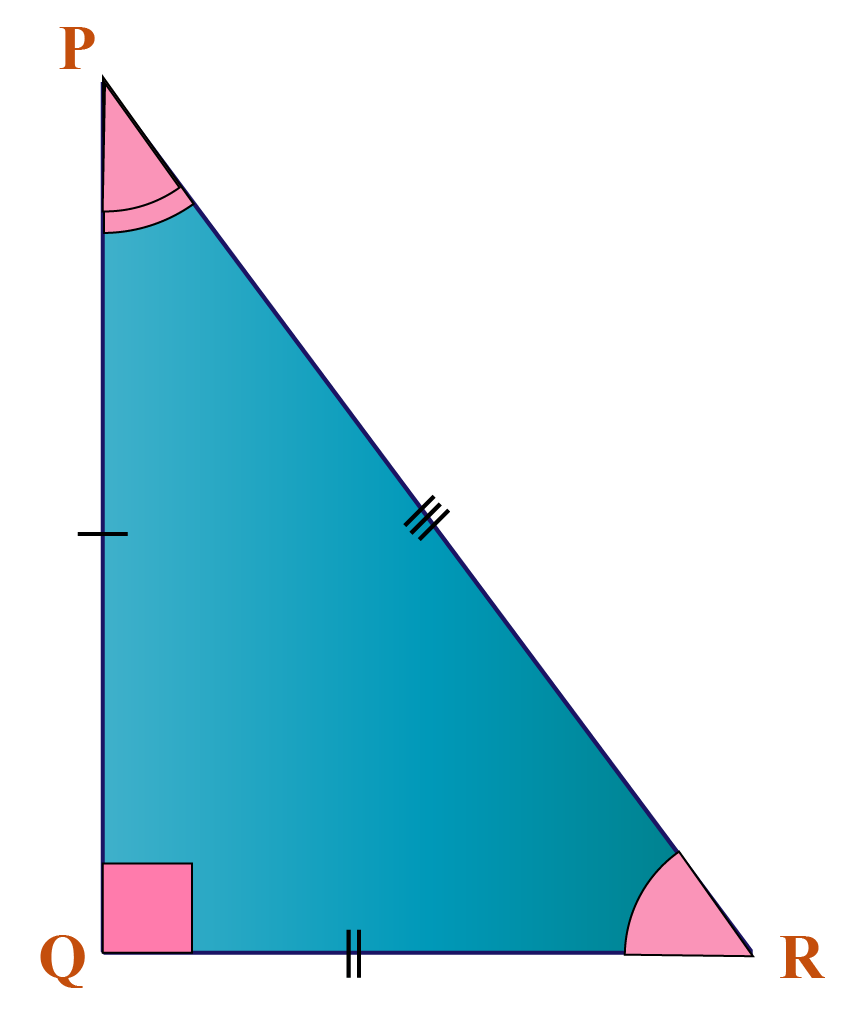### Obtuse Scalene Triangle

A triangle which has one vertex angle as obtuse and all 3 sides measure different is called an obtuse scalene triangle.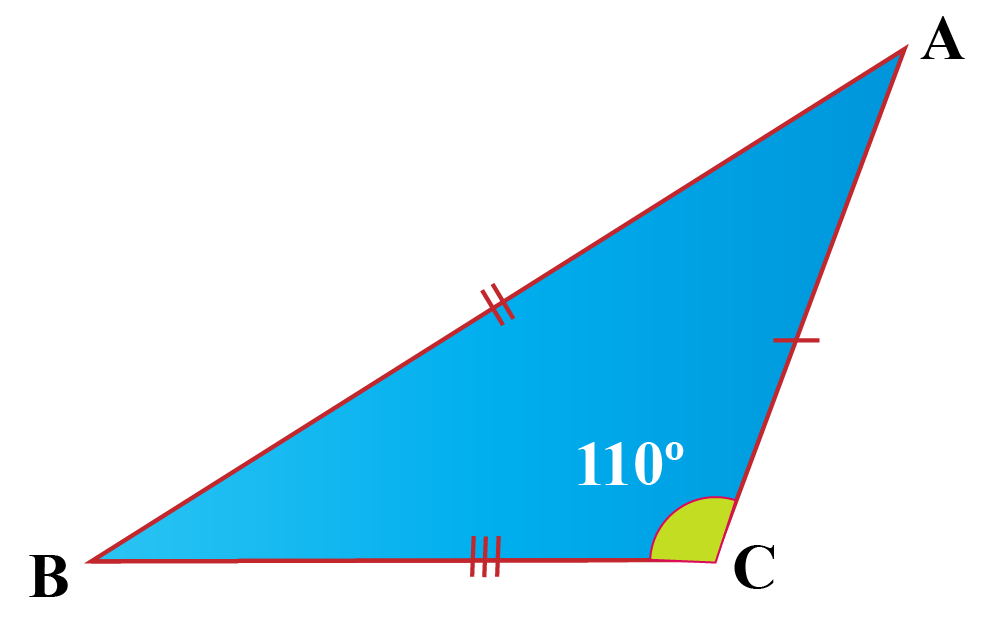### Acute Scalene Triangle

A triangle which has 3 unequal sides and all angles are acute angles is called an acute scalene triangle.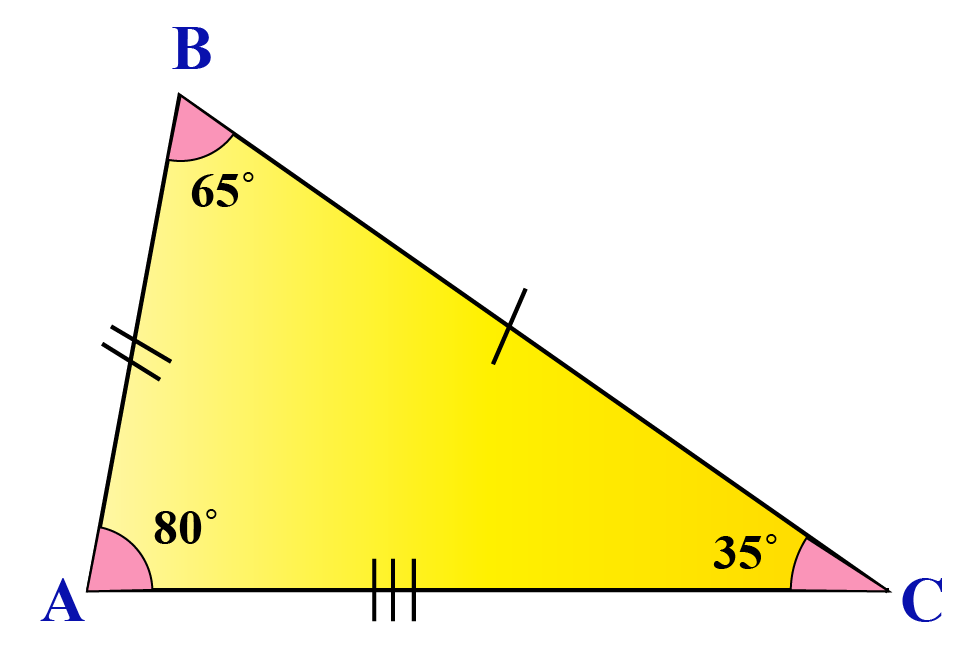Drag the vertices in the simulation below to understand the various kinds of triangles and visually observe the relation between the elements of the triangle.Think Tank
1. Can a triangle have more than 1 obtuse angle?
2. Is it possible to construct an obtuse-angled equilateral triangle?
3. Can a triangle have two right angles?
4. Can all the 3 angles of a triangle be less than 60 $$^\circ$$?

Help your child score higher with Cuemath’s proprietary FREE Diagnostic Test. Get access to detailed reports, customised learning plans and a FREE counselling session. Attempt the test now.

## Solved Examples & Problems

Improve your knowledge on the different types of triangles with examples from our experts.

 Example 1

In the given triangle PQR, identify the following:

1. Angle opposite to the side RQ
2. Side opposite to $$\angle \text{P}$$Solution: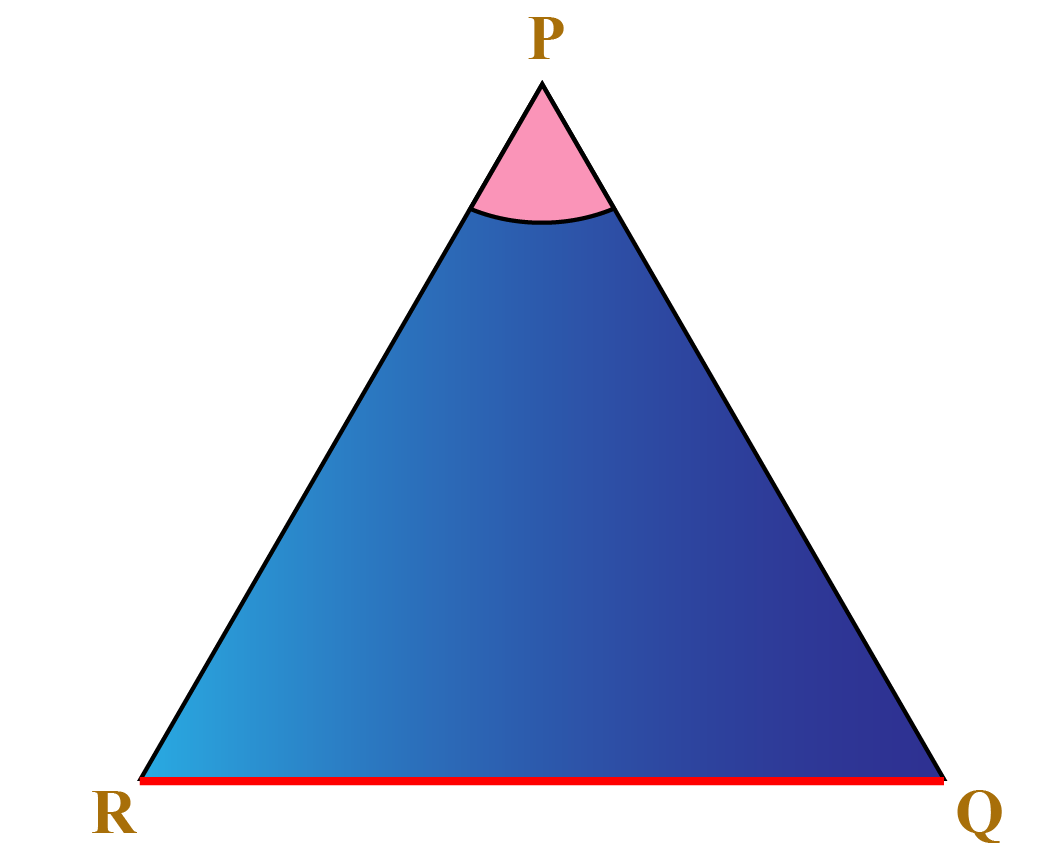In $$\Delta \text{PQR}$$, Angle opposite to side RQ is $$\angle \text{P}$$ and the side opposite to $$\angle \text{P}$$ is RQ

 Example 2

Name the type of the triangle which has the following elements:

$$\Delta \text{ABC}$$ with sides of length AB = 7 cm, BC = 8 cm and AC = 9 cm.

Solution:

All the 3 sides of this triangle measure different.

Thus, it is a scalene triangle.$$\therefore \Delta \text{ABC}$$ is a Scalene Triangle
 Example 3

What type of triangle is $$\Delta$$XYZ if  $$\angle \text{Y}$$= 90° and XY = YZ?

Solution:

Since $$\angle \text{Y}$$= 90°, it is a right triangle.

XY = YZ means 2 sides of the triangle are equal.

This indicates that $$\Delta$$XYZ is also an isosceles triangle.$$\therefore\Delta \text{XYZ}$$ is an Isosceles Right Triangle
 Example 4

Tina bought a pair of earrings and Roxy said, "Triangle earrings! I like them."

Look at Tina's earrings.

Is Roxy correct in identifying them as triangles?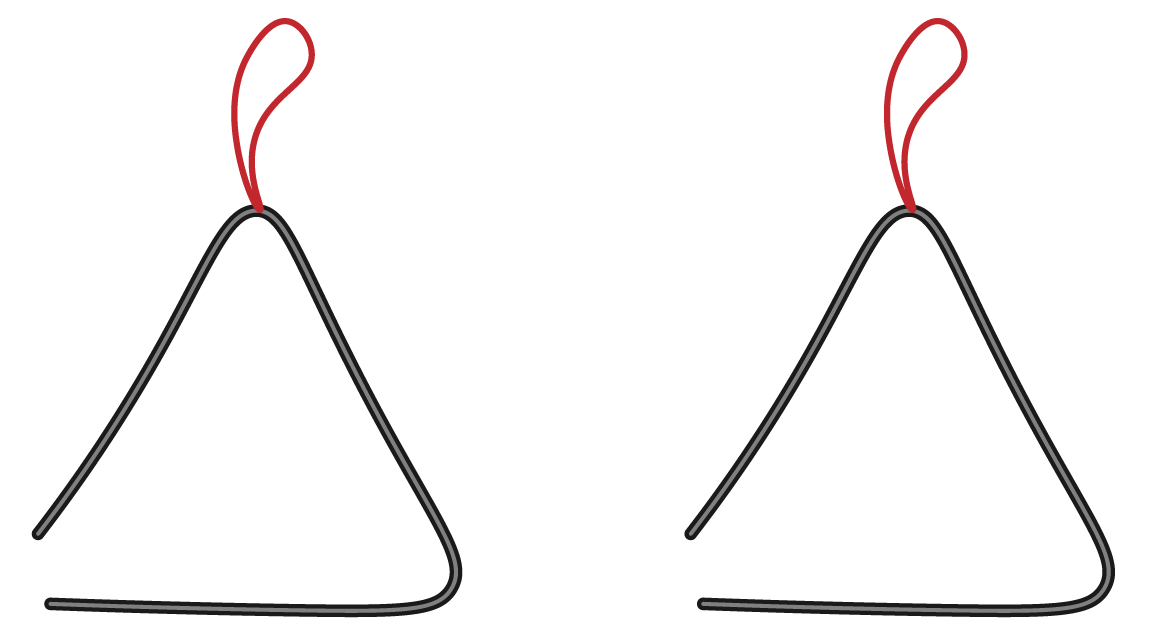Solution:

Though each pair of the earrings have 3 sides, it is not a closed shape.

Hence, we cannot call it a triangle.

A triangle is a closed shape made with 3 line segments.

 $$\therefore$$ Roxy is wrong; they are not triangles
 Example 5

Can a triangle have 72°, 100° and 30° as its vertex angles?

Solution:

The sum of the angles of a triangle is 180°

72° + 100° + 30° =  202°

which is more than 180°

We cannot have a triangle with 72°, 100° and 30° as its vertex angles.

 $$\therefore$$ We can't have a triangle with these measures.Challenging Questions

 1. Count the number of triangles in each figure.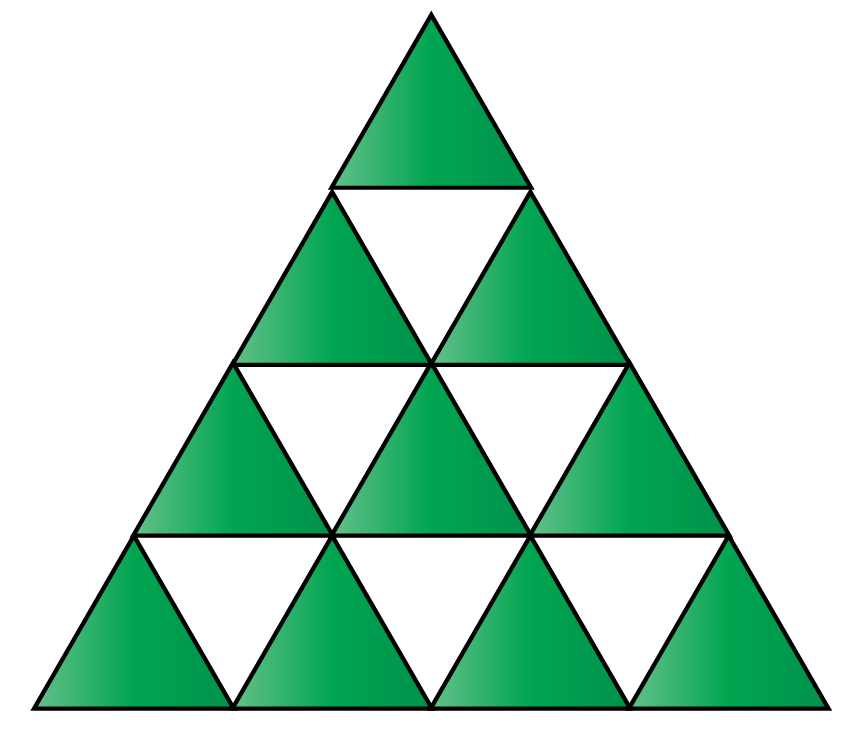CLUEless in Math? Check out how CUEMATH Teachers will explain Types of Triangles to your kid using interactive simulations & worksheets so they never have to memorise anything in Math again!

Explore Cuemath Live, Interactive & Personalised Online Classes to make your kid a Math Expert. Book a FREE trial class today!

## Practice Questions

Here are a few activities for you to practice.

IMO (International Maths Olympiad) is a competitive exam in Mathematics conducted annually for school students. It encourages children to develop their math solving skills from a competition perspective.

## 1. What are the 7 types of triangles?

The 7 different types of triangles are equilateral triangle, right isosceles triangle, obtuse isosceles triangle, acute isosceles triangle, right scalene triangle, obtuse scalene triangle and acute scalene triangle.

## 2. What are the different types of triangles based on sides?

Scalene triangle, Equilateral triangle and Isosceles triangle are the 3 types of triangles based on sides.

## 3. What are the different types of triangles based on angles?

Right-angled triangle, Acute-angled triangle and Obtuse-angled triangle are the 3 types of triangles based on angles.

More Important Topics
Numbers
Algebra
Geometry
Measurement
Money
Data
Trigonometry
Calculus